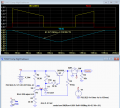# Low battery level shutdown circuit to protect lithium batteries from deep discharge?

#### Mahonroy

Joined Oct 21, 2014
315
Hello, I have a boosting voltage regulator with an "enable" pin on it, and I am using lithium ion batteries which have a voltage between 2.7v to 4.2 volt (3.8 volt nominal). If the "enable" pin is pulled high to the battery level, the voltage regulator starts boosting the voltage and regulating the voltage. If the "enable" pin is pulled low to ground, the voltage regulator shuts down, and has a mosfet that does a complete load disconnect. I have a power switch connected up to this "enable" pin to turn it on and off.

It is recommended to never let the lithium ion batteries discharge below 2.7 volts. Do you know of a circuit that I could also incorporate into this enable pin so that if the voltage is below 2.7v, it will keep the "enable" pin low and prevent it from powering up - regardless if the power switch is turned on or off?

I'm imagining a circuit that outputs low when the voltage is below 2.7v, and outputs high when the voltage is above 2.7v... and then using an AND gate to combine this signal along with the power button signal? Is there a better way of accomplishing this?

Thanks and any help or advice is greatly appreciated!

#### Mahonroy

Joined Oct 21, 2014
315
I stumbled across this circuit where they mention if IN falls below 1.76V the output goes to 0V, and if the input rises above 1.94V the output goes to 3.3V. I figured I could tweak it to fit my needs...... however, when I try to simulate the circuit it doesn't work right. I'm wondering if this is even correct or if I'm missing something?#### TeeKay6

Joined Apr 20, 2019
572
I stumbled across this circuit where they mention if IN falls below 1.76V the output goes to 0V, and if the input rises above 1.94V the output goes to 3.3V. I figured I could tweak it to fit my needs...... however, when I try to simulate the circuit it doesn't work right. I'm wondering if this is even correct or if I'm missing something?The logic is inverted from what you state. If IN < 1.76V, OUT=high; if IN > 1.94V, OUT=low. (N.B. I have not checked that 1.76V/1.94V are the correct trigger levels.) This is an example of a class of circuits called "comparators" or "level detectors". This particular example uses "hysteresis" provided by the positive feedback through R3. For example, once IN has gone below 1.76V, the trigger level will be changed to 1.94V (with OUT=high=3.3V). In order to make OUT go low (0V) again, IN must rise above the new trigger level (1.94V).

#### Mahonroy

Joined Oct 21, 2014
315
So this requires a voltage higher than the battery in order to work. To detect the voltage of 2.7v, but also being powered by this same voltage (4.2v to 2.7v) how could that work? I would power the op amp directly by the battery, would I then need to apply a 2.7 volt reference to the "-" of the op amp, and let the battery voltage fall to trigger it?

#### TeeKay6

Joined Apr 20, 2019
572
So this requires a voltage higher than the battery in order to work. To detect the voltage of 2.7v, but also being powered by this same voltage (4.2v to 2.7v) how could that work? I would power the op amp directly by the battery, would I then need to apply a 2.7 volt reference to the "-" of the op amp, and let the battery voltage fall to trigger it?
It is not clear to me what is your overall scheme. You speak of a battery and a "boost circuit with enable input". Is the intent of the boost circuit to power some circuitry that requires more than the discharged battery voltage (2.7V)? I assume that an acceptable solution would disable the boost when the battery voltage dropped to 2.7V and the boost would remain disabled until you somehow recharged the battery?

#### crutschow

Joined Mar 14, 2008
25,377
Here's the LTspice simulation of a circuit using a TLV431 voltage reference as a comparator.
The output is high when the TLV431 Ref pin voltage, as determined by the voltage divider, is above 1.24V, and low when it is below 1.24V.
The Enable (yellow trace) is thus high only when the battery voltage (blue trace) is above 2.7V (for the given resistor values and pot setting) and the enable switch (red trace), SW1 is on.
Pot U2 adjusts the trip voltage.
R6 adds a small amount of positive feedback to give about 100mV of hysteresis between the negative going and positive going trip points to minimize any oscillations when the circuit changes states.